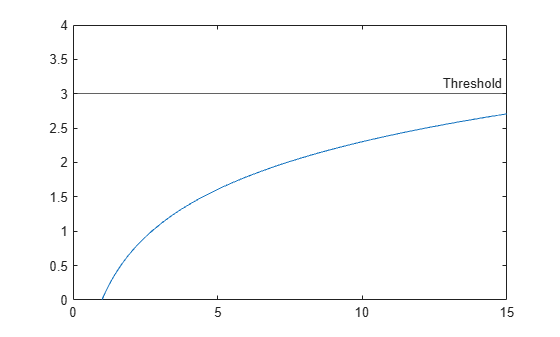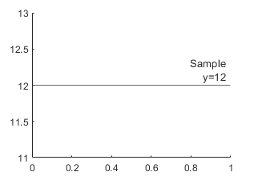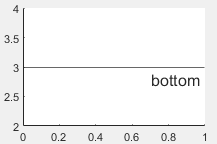# yline

Horizontal line with constant y-value

## Syntax

``yline(y)``
``yline(y,LineSpec)``
``yline(y,LineSpec,labels)``
``yline(___,Name,Value)``
``yline(ax,___)``
``yl = yline(___)``

## Description

example

````yline(y)` creates a horizontal line at one or more y-coordinates in the current axes. For example, `yline(2)` creates a line at `y=2`.```

example

````yline(y,LineSpec)` specifies the line style, the line color, or both. For example, ```xline([12 20 33],'--b')``` creates three dashed blue lines.```

example

````yline(y,LineSpec,labels)` adds labels to the lines.```

example

````yline(___,Name,Value)` specifies constant line properties using one or more name-value pair arguments. For a list of properties, see ConstantLine Properties. Specify name-value pairs after all other input arguments.```

example

````yline(ax,___)` creates the line in the specified axes instead of the current axes. To determine the current axes use `gca`. ```

example

````yl = yline(___)` returns a `ConstantLine` object or an array of `ConstantLine` objects. Use `yl` to modify or query properties of the line after it is created.```

## Examples

collapse all

Create a horizontal line at y = 2.5.

`yline(2.5);`To create a line with a label, you must also specify the line style. The default line style is a solid line, `'-'`.

```fplot(@(x) log(x)); xlim([0 15]); ylim([0 4]); yline(3,'-','Threshold');```Define a set of `x` and `y` vectors and plot them. Then pad the y-axis with extra space above and below the maximum and minimum plotted values.

```x = 0:0.1:60; y = 4.*cos(x)./(x+2); plot(x,y) ylim padded```Find the maximum and minimum values of `y`. Create horizontal lines at those locations with the labels `'Max'` and `'Min'`.

```ymax = max(y); ymin = min(y); yline([ymax ymin],'--',{'Max','Min'})```Create a blue dash-dot line at y = 4.

`yline(4,'-.b');`Create a horizontal dashed line with a label and a specified line width.

```x = -2:0.25:2; [X,Y] = meshgrid(x); Z = X.*exp(-X.^2-Y.^2); contour(X,Y,Z,30) yl = yline(0,'--','y = 0','LineWidth',3);```Change the horizontal alignment of the label and the color of the line.

```yl.LabelHorizontalAlignment = 'center'; yl.Color = [.80 0 .40];```Call the `tiledlayout` function to create a 1-by-2 tiled chart layout. Call the `nexttile` function to create the axes objects `ax1` and `ax2`. Then plot into each of the axes. Add a horizontal line to the first plot by passing `ax1` to the `yline` function.

```tiledlayout(1,2) ax1 = nexttile; x = -pi/2:pi/60:pi/2; y1 = tan(sin(x)) + cos(sin(x)); plot(ax1,x,y1) ax2 = nexttile; x = -pi/2:pi/60:pi/2; y2 = tan(cos(x)) + exp(x); plot(ax2,x,y2) yline(ax1,1.0);```## Input Arguments

collapse all

y-coordinates, specified as a scalar or a vector containing numeric, categorical, datetime, or duration values. The data type of `y` must match the data type of the y-axis.

Example: `yline(10)` displays a line at `y=10`.

Example: `yline([13 20 33])` displays lines at `y=13`, `y=20`, and `y=33`.

Data Types: `single` | `double` | `int8` | `int16` | `int32` | `int64` | `uint8` | `uint16` | `uint32` | `uint64` | `logical` | `categorical` | `datetime` | `duration`

Line style, marker, and color, specified as a character vector or string containing symbols. The symbols can appear in any order. You do not need to specify all three characteristics (line style, marker, and color). For example, if you omit the line style and specify the marker, then the plot shows only the marker and no line.

Example: `'--or'` is a red dashed line with circle markers

Line StyleDescription
`-`Solid line
`--`Dashed line
`:`Dotted line
`-.`Dash-dot line
MarkerDescription
`'o'`Circle
`'+'`Plus sign
`'*'`Asterisk
`'.'`Point
`'x'`Cross
`'_'`Horizontal line
`'|'`Vertical line
`'s'`Square
`'d'`Diamond
`'^'`Upward-pointing triangle
`'v'`Downward-pointing triangle
`'>'`Right-pointing triangle
`'<'`Left-pointing triangle
`'p'`Pentagram
`'h'`Hexagram
ColorDescription

`y`

yellow

`m`

magenta

`c`

cyan

`r`

red

`g`

green

`b`

blue

`w`

white

`k`

black

Target axes, specified as an `Axes` object. Use this argument if you want `yline` to plot in axes other than the current axes.

Line labels, specified as a character vector, cell array of character vectors, string array, or numeric array. To create a multiline label, use a string array or a cell array of character vectors.

#### Create a Label for One Line

Specify a character vector or a string scalar to display one line of text. To display multiple lines of text, specify a cell array of character vectors or a string array.

PresentationHow to Specify `labels`Example
One line of textSpecify a character vector or a string scalar.

`yline(12,'-','Sample')`Multiline textSpecify a cell array of character vectors or a string array. Each element in the array is a different line of text.
`yline(12,'-',{'Sample','x=12'})`#### Create Labels for Multiple Lines

Specify a character vector or a string scalar to display the same label next to all the lines. Specify a cell array of character vectors or a string array to display different labels for each line.

PresentationHow to Specify `labels`Example
One shared text labelSpecify a character vector or a string scalar.

`yline([13 20 33],'-','Sample')`Different labels for each lineSpecify a cell array of character vectors or a string array. Each element in the array is a label for a different line. The number of elements in the `labels` array must match the length of `x`.
```label = {'Sample 1','Sample 2','Sample 3'}; yline([13 20 33],'-',label)```Different labels with some multiline labelsCreate a cell array of character vectors or a string array. Use the `sprintf` function to insert newline characters (`'\n'`) in the text.
```label = {'Sample 1',sprintf('Sample 2\nx=20'),'Sample 3'}; yline([13 20 33],'-',label)```### Name-Value Pair Arguments

Specify optional comma-separated pairs of `Name,Value` arguments. `Name` is the argument name and `Value` is the corresponding value. `Name` must appear inside quotes. You can specify several name and value pair arguments in any order as `Name1,Value1,...,NameN,ValueN`.

Example: ```yline(3,':','Median Price','LabelHorizontalAlignment','center')``` specifies the horizontal alignment of the label as `'center'`.

Note

The properties listed here are only a subset. For a complete list, see ConstantLine Properties.

Line color, specified as an RGB triplet, a hexadecimal color code, a color name, or a short name.

For a custom color, specify an RGB triplet or a hexadecimal color code.

• An RGB triplet is a three-element row vector whose elements specify the intensities of the red, green, and blue components of the color. The intensities must be in the range `[0,1]`; for example, ```[0.4 0.6 0.7]```.

• A hexadecimal color code is a character vector or a string scalar that starts with a hash symbol (`#`) followed by three or six hexadecimal digits, which can range from `0` to `F`. The values are not case sensitive. Thus, the color codes `'#FF8800'`, `'#ff8800'`, `'#F80'`, and `'#f80'` are equivalent.

Alternatively, you can specify some common colors by name. This table lists the named color options, the equivalent RGB triplets, and hexadecimal color codes.

Color NameShort NameRGB TripletHexadecimal Color CodeAppearance
`'red'``'r'``[1 0 0]``'#FF0000'``'green'``'g'``[0 1 0]``'#00FF00'``'blue'``'b'``[0 0 1]``'#0000FF'``'cyan'` `'c'``[0 1 1]``'#00FFFF'``'magenta'``'m'``[1 0 1]``'#FF00FF'``'yellow'``'y'``[1 1 0]``'#FFFF00'``'black'``'k'``[0 0 0]``'#000000'``'white'``'w'``[1 1 1]``'#FFFFFF'``'none'`Not applicableNot applicableNot applicableNo color

Here are the RGB triplets and hexadecimal color codes for the default colors MATLAB® uses in many types of plots.

`[0 0.4470 0.7410]``'#0072BD'``[0.8500 0.3250 0.0980]``'#D95319'``[0.9290 0.6940 0.1250]``'#EDB120'``[0.4940 0.1840 0.5560]``'#7E2F8E'``[0.4660 0.6740 0.1880]``'#77AC30'``[0.3010 0.7450 0.9330]``'#4DBEEE'``[0.6350 0.0780 0.1840]``'#A2142F'`Example: `'g'`

Example: `[0.6 0.2 0.5]`

Example: `'#D2F9A7'`

Line width, specified as a positive value in points.

Legend label, specified as a character vector or string scalar. The legend does not display until you call the `legend` command. If you do not specify the text, then `legend` sets the label using the form `'dataN'`.

Horizontal alignment of the label with respect to the line, specified as one of the options in the table.

OptionDescriptionExample
`'right'`Right side of the line`'left'`Left side of the line`'center'`Center of the lineVertical alignment of the label with respect to the line, specified as one of the options in the table.

OptionDescriptionExample
`'top'`Top of the line.`'middle'`Middle of the line. The label segments the line.`'bottom'`Bottom of the line.Label orientation, specified as `'aligned'` or `'horizontal'`. Examples are shown in the table.

OrientationDescriptionExample
`'aligned'`Label has the same orientation as the specified line.`'horizontal'`Label is horizontal, regardless of the line orientation.## Algorithms

In a 3-D view of the axes, the constant line appears in the x-y plane at the midpoint of the z-axis limits. If the axes are rotated, then the constant line rotates accordingly.

### Properties

Introduced in R2018b